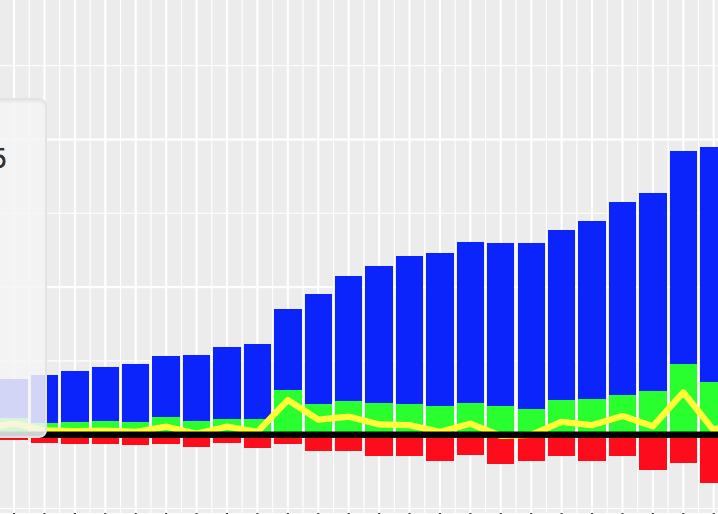2 views

I am using the following code to plot a bar-chart:

import matplotlib.pyplot as pls my_df.plot(x='my_timestampe', y='col_A', kind='bar') plt.show()

The plot works fine. However, I want to improve the graph by having 3 columns: 'col_A', 'col_B', and 'col_C' all on the plot. Like in the example figure below:I would like the col_A displayed in the blue above x-axis, col_B in red below x-axis, and col_C in the green above the x-axis. Is this something possible in matplotlib? How do I make changes to plot all the three columns? Thanks!

by (108k points)

As you know that the v0.21.0rc1 gives a warning

UserWarning: Pandas doesn't allow columns to be created via a new attribute name

Instead, what you can do is

df[["X", "A", "B", "C"]].plot(x="X", kind="bar")